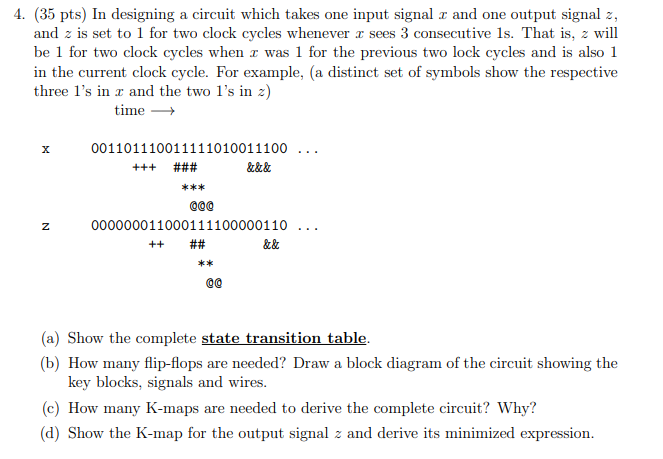# (Solved): PLEASE SHOW HOW YOU DID A B C and D 4. (35 pts) In designing a circuit which takes one input signa ...PLEASE SHOW HOW YOU DID A B C and D

4. (35 pts) In designing a circuit which takes one input signal \( x \) and one output signal \( z \), and \( z \) is set to 1 for two clock cycles whenever \( x \) sees 3 consecutive 1s. That is, \( z \) will be 1 for two clock cycles when \( x \) was 1 for the previous two lock cycles and is also 1 in the current clock cycle. For example, (a distinct set of symbols show the respective three 1's in \( x \) and the two 1's in \( z \) ) time \( \longrightarrow \) (a) Show the complete state transition table. (b) How many flip-flops are needed? Draw a block diagram of the circuit showing the key blocks, signals and wires. (c) How many K-maps are needed to derive the complete circuit? Why? (d) Show the K-map for the output signal \( z \) and derive its minimized expression.

We have an Answer from Expert